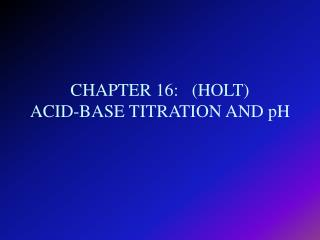DownloadDownload PresentationCHAPTER 16: (HOLT) ACID-BASE TITRATION AND pH

# CHAPTER 16: (HOLT) ACID-BASE TITRATION AND pH

Télécharger la présentation## CHAPTER 16: (HOLT) ACID-BASE TITRATION AND pH

- - - - - - - - - - - - - - - - - - - - - - - - - - - E N D - - - - - - - - - - - - - - - - - - - - - - - - - - -
##### Presentation Transcript

1. CHAPTER 16: (HOLT)ACID-BASE TITRATION AND pH

2. I. Concentration Units for Acids and Bases • A. Chemical Equivalents • 1. Definition: quantities of solutes that have equivalent combining capacity • a. Acid: mass of one equivalent is numerically equal to the mass of one mole of the acid divided by the number of protons(H+ or H3O +) that one mole of the acid can provide • Example: • HCl 36 g/mol; 1 eq = 1H +; 36 g/mol H + • H2SO4 98g/mol; 2 eq = 2H +; 49 g/mol H +

3. B. Base: mass of one equivalent is numerically equal to the mass of one mole of the base divided by the number of protons(OH-) that one mole of the base can provide • Example: • NaOH 40 g/mol; 1 eq = 1 OH-; 40 g/mol OH- • Ca(OH)2 74 g/mol; 2 eq = 2 OH-; 37 g/mol OH-

4. B. Normality • Definition: number of equivalents of solute per liter of solution • N = eq of solute • L of solution

5. C. Relationship Between Normality and Molarity • N = nM • N: Normality • n: number of equivalents (# of H+= or OH-) • M: Molarity • Example: • 1M HCl = 1N HCl 1M NaOH = 1N NaOH • 1M H2SO4 = 2N H2SO4 1M Ca(OH)2 = 2N Ca(OH)2

6. II. Aqueous Solutions and the Concept of pH • A. Self-Ionization of Water • 1. Definition: Two water molecules interact to produce a hydronium ion and a hydroxide ion by proton transfer - forms a weak electrolyte • 2. [ ] is symbol used to indicate concentration in moles per liter (Molarity) • 3. H2O + H2O <---> H3O+ + OH- ; • in pure water [H3O+ ] = [OH- ] • 4. [H3O+ ][OH- ] = 10-14 • 5. If the [H3O+ ] increases then the [OH- ] decreases or • If the [H3O+ ] decreases then the [OH- ] increases

7. B. The pH scale • 1. pH -- the negative of the common logarithm of the hydronium ion concentration • pH = -log[H3O+ ] • 2. Acid: pH < 7 • 3. Base: pH > 7 • 4. Neutral: pH = 7

8. C. Calculations involving pH • pH = -log[H3O+ ] • 0.001 M HCl = [H3O+ ] =1 x 10 -3 • pH = -log[1 x 10-3] • pH = 3 (acid) • {Remember that [H3O+ ][OH- ] = 1 x 10-14 ; so if [H3O+ ] = 1 x 10-3; then [OH- ] = 1 x 10-11 • FYI: there is also pOH = - log[OH- ] and • pH + pOH = 14

9. III. Acid-Base Titrations • A. Indicators • 1. Definitions: • a. indicators - weak acid or base dyes whose colors are sensitive to pH, or hydronium, concentration • b. transition interval - the pH range over which an indicator changes color

10. 2. Types of indicators • a. Change color at about pH 7 • b. Change color below pH 7 • c. Change color above pH 7

11. B. The Principle of Titration • Definitions: • 1. Titration - the controlled addition and measurement of the amount of a solution of known concentration that is required to react completely with a measured amount of a solution of unknown concentration • 2.Standard solution - a solution that contains a precisely known concentration of a solute

12. 3. Equivalence point - in a neutralization reaction, the point at which there are equivalent quantities of hydronium and hydroxide ions • 4. End point - the point in a titration where an indicator changes color • 5. Primary standard - a highly purified compound, when used in solution to check the concentration of the known solution in a titration

13. C. Molarity and Titration • 1. Determine the moles of acid (or base) from the standard solution used during titration • 2. From a balanced chemical equation, determine the ratio of moles of acid (base) to base (acid) • 3. Determine the moles of solute of the unknown solution used during the titration • 4. Determine the molarity of the unknown solution

14. D. Normality and Titrations • Va x Na = Vb x Nb • Va : volume of the acid • Na : normality of the acid • Vb: volume of the base • Nb: normality of the base